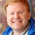Friday, May 1, 2015

Hypothesis testing is only mostly useless

UPDATE May 4: Nature recently reported the outcome of an attempt to replicate 100 results from psychology research. They characterize the findings as "worrying."

In my previous article I argued that classical statistical inference is only mostly wrong. About null-hypothesis significance testing (NHST),  I wrote:

“If the p-value is small, you can conclude that the fourth possibility is unlikely, and infer that the other three possibilities are more likely.”

Where “the fourth possibility” I referred to was:

“The apparent effect might be due to chance; that is, the difference might appear in a random sample, but not in the general population.”

Several commenters chastised me; for example:

“All a p-value tells you is the probability of your data (or data more extreme) given the null is true. They say nothing about whether your data is ‘due to chance.’”

My correspondent is partly right.  The p-value is the probability of the apparent effect under the null hypothesis, which is not the same thing as the probability we should assign to the null hypothesis after seeing the data.  But they are related to each other by Bayes’s theorem, which is why I said that you can use the first to make an inference about the second.

Let me explain by showing a few examples.  I’ll start with what I think is a common scenario:  suppose you are reading a scientific paper that reports a new relationship between two variables.  There are (at least) three explanations you might consider for this apparent effect:

A:  The effect might be actual; that is, it might exist in the unobserved population as well as the observed sample.
B:  The effect might be bogus, caused by errors like sampling bias and measurement error, or by fraud.
C:  The effect might be due to chance, appearing randomly in the sample but not in the population.

If we think of these as competing hypotheses to explain the apparent effect, we can use Bayes’s theorem to update our belief in each hypothesis.

Scenario #1

As a first scenario, suppose that the apparent effect is plausible, and the p-value is low.  The following table shows what the Bayesian update might look like:

 Prior Likelihood Posterior Actual 70 0.5–1.0 ~75 Bogus 20 0.5–1.0 ~25 Chance 10 p = 0.01 ~0.001

Since the apparent effect is plausible, I give it a prior probability of 70%.  I assign a prior of 20% to the hypothesis that the result is to due to error or fraud, and 10% to the hypothesis that it’s due to chance.  If you don’t agree with the numbers I chose, we’ll look at some alternatives soon.  Or feel free to plug in your own.

Now we compute the likelihood of the apparent effect under each hypothesis.  If the effect is real, it is quite likely to appear in the sample, so I figure the likelihood is between 50% and 100%.   And in the presence of error or fraud, I assume the apparent effect is also quite likely.

If the effect is due to chance, we can compute the likelihood directly.  The likelihood of the data under the null hypothesis is the p-value.  As an example, suppose p=0.01.

The table shows the resulting posterior probabilities.  The hypothesis that the effect is due to chance has been almost eliminated, and the other hypotheses are marginally more likely.  The hypothesis test helps a little, by ruling out one source of error, but it doesn't help a lot, because randomness was not the source of error I was most worried about.

Scenario #2

In the second scenario, suppose the p-value is low, again, but the apparent effect is less plausible.  In that case, I would assign a lower prior probability to Actual and a higher prior to Bogus.  I am still inclined to assign a low priority to Chance, simply because I don’t think it is the most common cause of scientific error.

 Prior Likelihood Posterior Actual 20 0.5–1.0 ~25 Bogus 70 0.5–1.0 ~75 Chance 10 p = 0.01 ~0.001

The results are pretty much the same as in Scenario #1: we can be reasonably confident that the result is not due to chance.  But again, the hypothesis test does not make a lot of difference in my belief about the validity of the paper.

I believe these examples cover a large majority of real-world scenarios, and in each case my claim holds up:  If the p-value is small, you can conclude that apparent effect is unlikely to be due to chance, and the other possibilities (Actual and Bogus) are more likely.

Scenario #3

I admit that there are situations where this conclusion would not be valid.  For example, if the effect is implausible and you have good reason to think that error and fraud are unlikely, you might start with a larger prior belief in Chance.  In that case a p-value like 0.01 might not be sufficient to rule out Chance:

 Prior Likelihood Posterior Actual 10 0.5 46 Bogus 10 0.5 46 Chance 80 p = 0.01 8

But even in this contrived scenario, the p-value has a substantial effect on our belief in the Chance hypothesis.  And a somewhat smaller p-value, like 0.001, would be sufficient to rule out Chance as a likely cause of error.

In summary, NHST is problematic but not useless.  If you think an apparent effect might be due to chance, choosing an appropriate null hypothesis and computing a p-value is a reasonable way to check.

1.1.2.